﻿ Calculating Numbers For Income Tax Return With Pen, Glasses And Calculator. Editorial Image - Image of accounting, money: 107759720

# Calculating numbers for income tax return with pen, glasses and calculator. Accounting, money.

## Calculating numbers for income tax return with pen, glasses and calculator

More similar stock images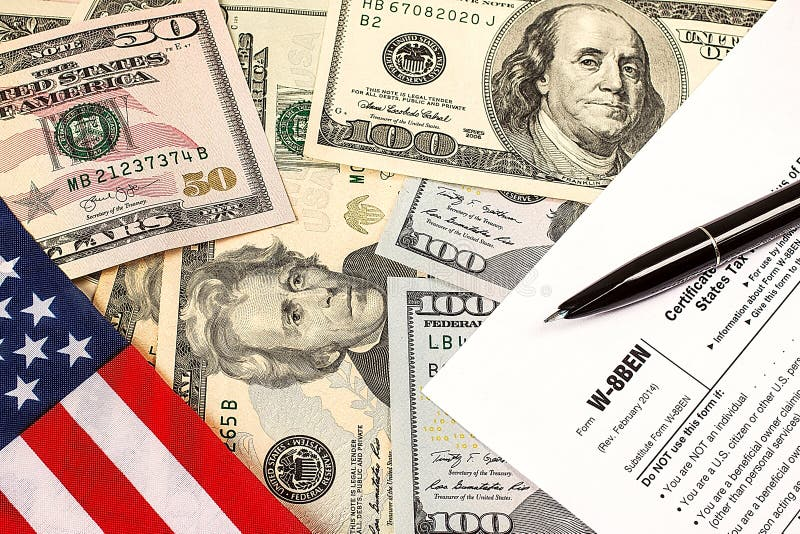Calculating numbers for income tax return with pen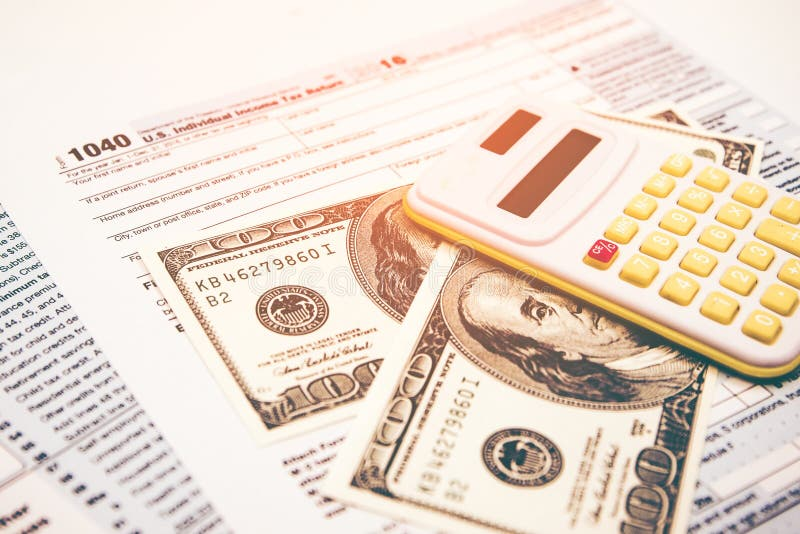Calculating numbers for income tax return.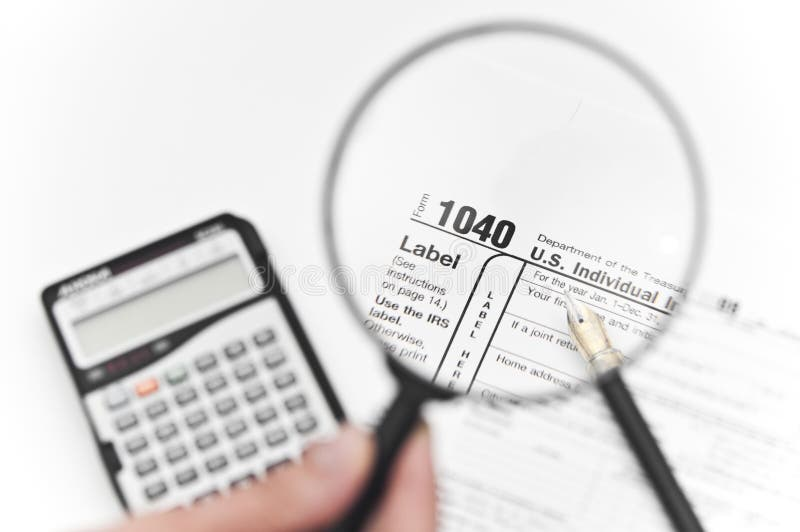Calculating taxes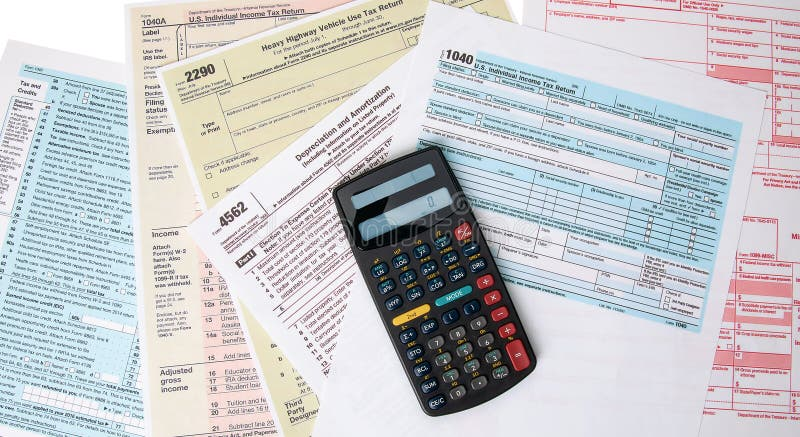Calculating Taxes Forms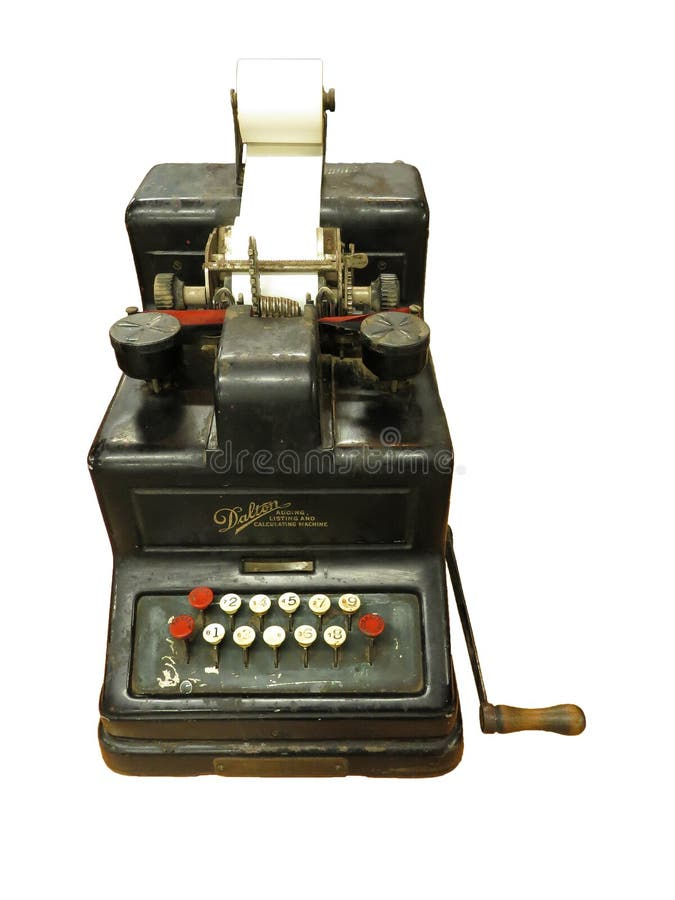Outline photo of old black calculator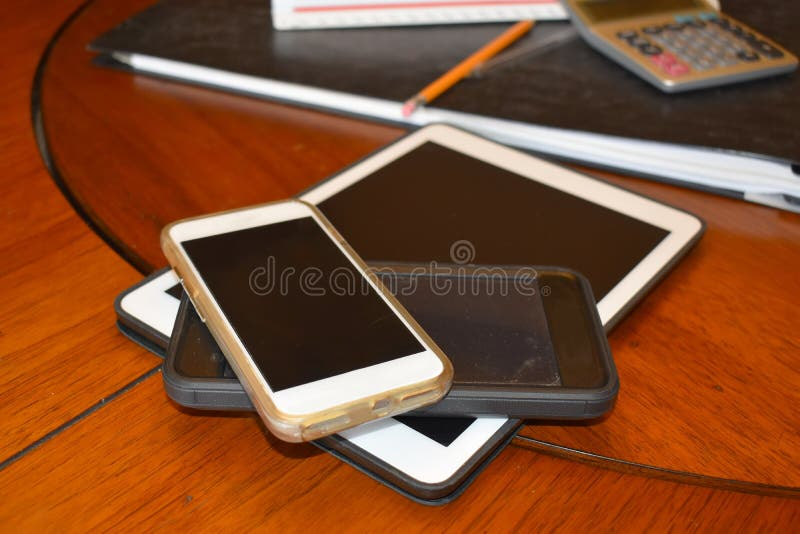Tablet and smart phones used in business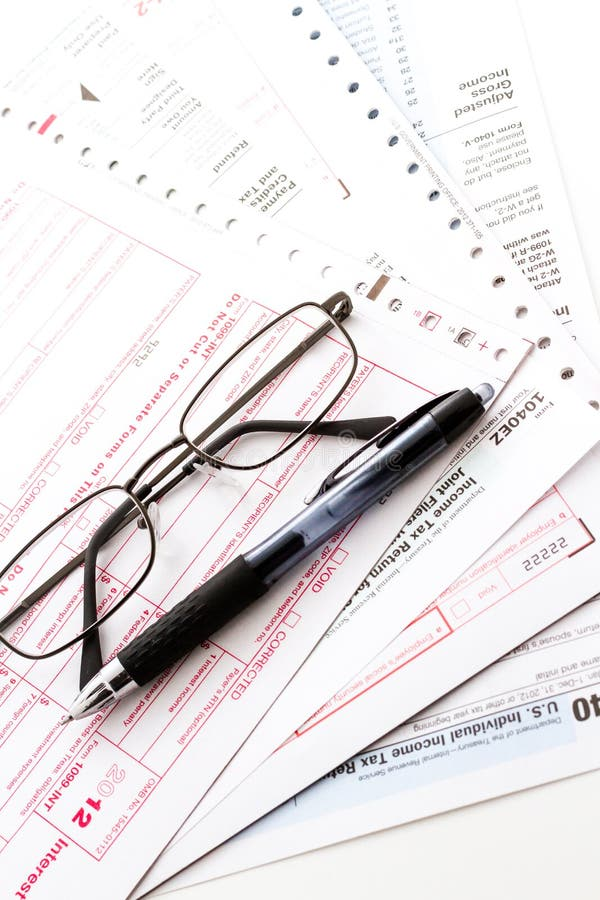Tax return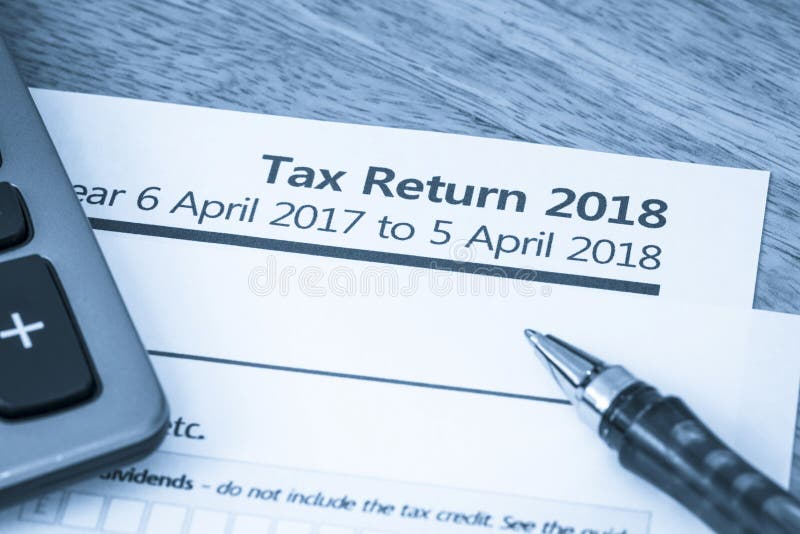Tax return form UK 2018Filling tax form
More stock photos from Maxopphoto's portfolio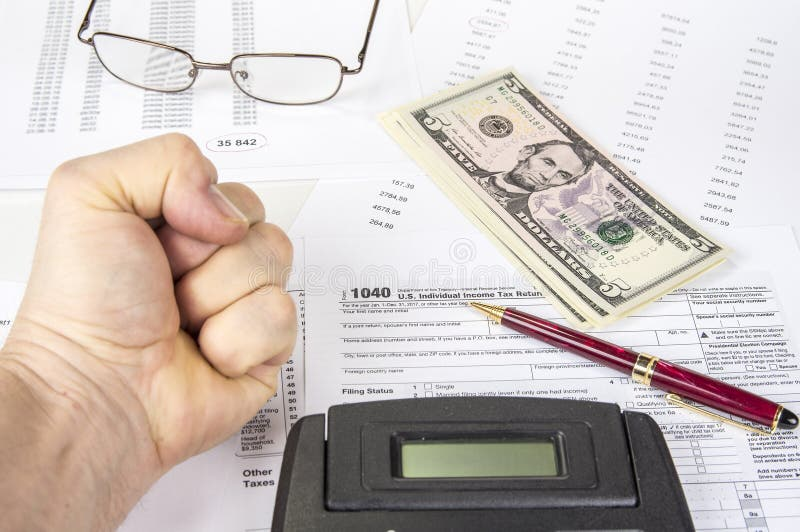Calculating numbers for income tax return with pen, glasses and calculator.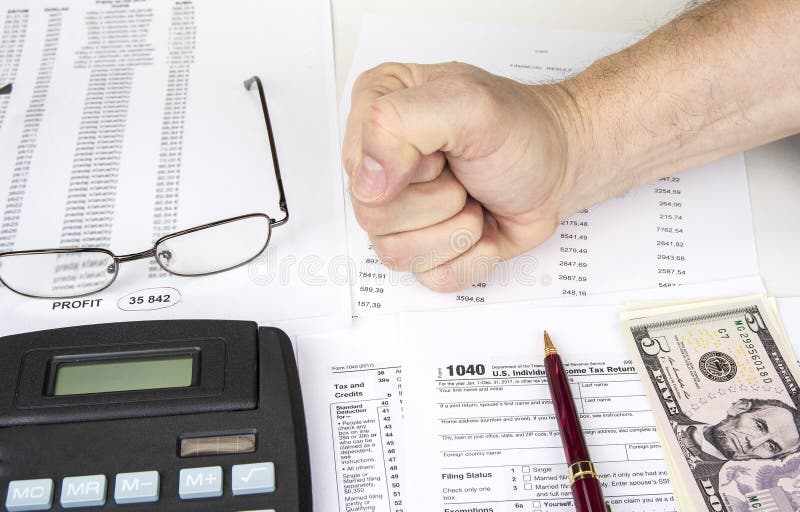Calculating numbers for income tax return with pen, glasses and calculator.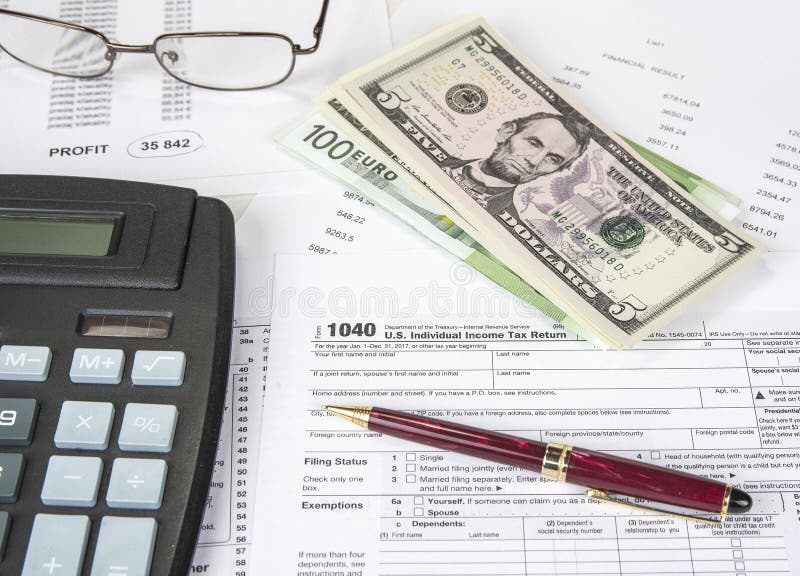Calculating numbers for income tax return with pen, glasses and calculator.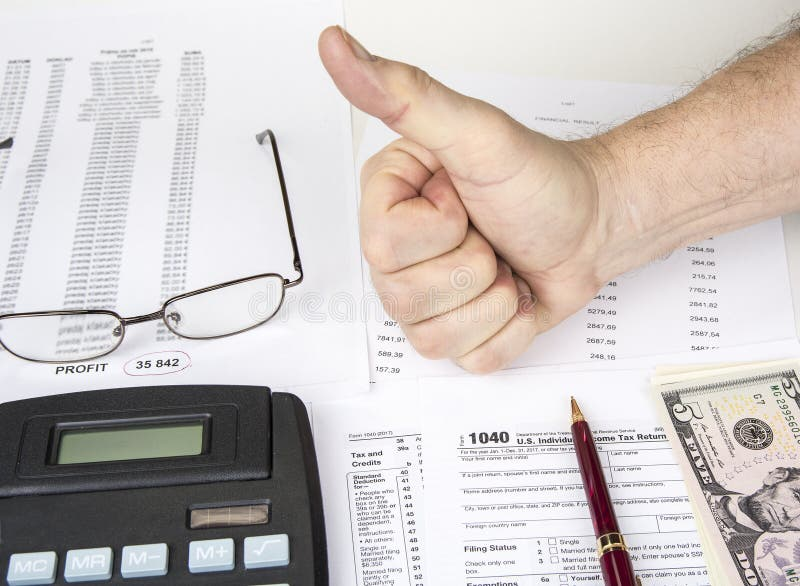Calculating numbers for income tax return with pen, glasses and calculator.
Related categories
Extended licenses